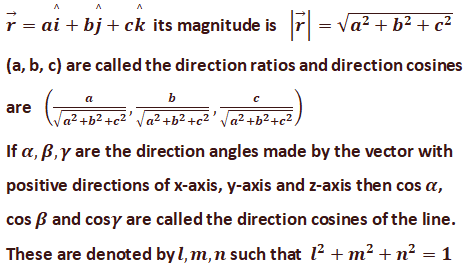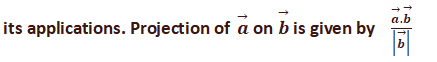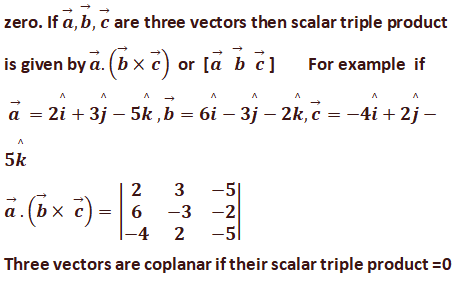### Common Errors in Secondary Mathematics

Common Errors Committed  by the  Students  in Secondary Mathematics   Errors  that students often make in doing secondary mathematics  during their practice and during the examinations  and their remedial measures are well explained here stp by step.  Some Common Errors in Mathematics

### Lesson Plan Math Class 12 Ch-10 | Vectors

E- LESSON PLAN   SUBJECT MATHEMATICS    CLASS 10+2
Lesson Plan, Class XII Subject Mathematics, chapter 10, vector, for Mathematics Teacher. Effective way of Teaching Mathematics. Top planning by the teacher for effective teaching in the class. E lesson planning for mathematics.
 Board – CBSE CLASS –XII SUBJECT- MATHEMATICS CHAPTER 10  :- Vectors

TOPIC:- Chapter : 10 : Vectors

DURATION:-

This chapter is divided into 13 modules and is completed in 15 class meetings.

PRE- REQUISITE KNOWLEDGE:-
Knowledge of simple concepts of trigonometry , geometry and algebra.
Knowledge of coordinate geometry class X.

TEACHING AIDS:-
Green Board, Chalk,  Duster, Charts, smart board, projector, laptop etc.

METHODOLOGY:-   Lecture method

OBJECTIVES:-
• Vector and Scalar quantities.
• Types of vectors (equal, unit, zero, negative, parallel and collinear vectors)
• Position vector of a point and components of a vector.
• Magnitude and direction of a vector.
• Direction ratios and direction cosines of a vector.
• Addition of vectors, multiplication of a vector by a scalar.
• Position vector of a point dividing a line segment in a given ratio(section formula with internal and external division and mid-point formula.
• Properties and applications of scalar(dot) product of two vectors, vector(cross) product of two vectors and scalar triple product of three vectors.

PROCEDURE :-
Start the session by asking the questions related to the quantities around us and start differentiating the quantities whether they have magnitude or direction or both. Now introduce the topic vector step by step as follows.

S.No.

## TOPICS AND EXPLANATION1

Introduction of vectors, definition of scalar and vector quantities and making the different lists of scalar and vector quantities around us.

Scalar: The quantities which has only magnitude are called scalar quantities, for example distance.

Vector: The quantities which has  magnitude and direction both are called vector quantities, for example displacement.

2

Explain the different types of vectors, like equal, unit, zero, negative, parallel , co-initial and collinear vectors. Give their definitions with examples.3

Explain the method of finding the position vector of a point, by giving some examples and explain the method of finding the magnitude of a vector.

4

Define direction angles, cosines and direction ratios. Explain the concept  by taking an example of a general vector.

Direction angles: These are the angles made by the vector with the positive direction of the axis.

Direction cosines: cosines of the direction angles are called direction cosines.

Direction ratios: The terms which are proportional to the direction cosines are called direction ratios.5

Explain the triangle law and parallelogram law of vector addition, also explain the different properties of vector addition.

6

Explain the method of multiplication of a vector with a scalar.

7

Explain the components of vectors along x-axis, y-axis, z-axis and explain the formation of the vector in terms of components.

8

Explain the method of finding a vector joining two points.9

Explain the section formula for internal and external division and then explain the mid-point formula.

10

Product of two vectors : Scalar or dot product of two vectors and their important properties.11

Explain the concept of projection of a vector on a line and12

Explain the method of finding the area of triangle and parallelogram by using vector algebra. Also explain the Lagrange’s identities.13

Explain the scalar triple product of three vector.

Three vectors are coplanar if their scalar triple product isEXPECTED OUTCOMES:-
After studying this lesson students should know
• scalar and vector quantities.
• different types  of vectors, their components, their magnitude.
• scalar product of two vectors
• vector product of two vectors
• Scalar triple product of three vectors.
• Students should know the method of finding the area of triangle and area of parallelogram by using vectors.
• Students also be able to apply the section formula, mid-point formula and projection formula in different problems.
STUDENTS DELIVERABLES:-
• Review questions given by the teacher.
• Students should prepare the presentation on the scalar product, cross product and scalar triple product of three vectors and their applications in different problems.
• Solve NCERT problems with examples.
EXTENDED LEARNING:-
Students can extend their learning in Mathematics through the RESOURCE CENTRE.  Students can also find many interesting topics on mathematics at cbsemathematics.com

ASSESSMENT TECHNIQUES:-
• Assignment sheet will be given as home work at the end of the topic.
• Separate sheets which will include questions of logical thinking and Higher order thinking skills will be given to the above average students.
• Class Test , Oral Test , worksheet and Assignments. can be made the part of assessment.
• Re-test(s) will be conducted on the basis of the performance of the students in the test.

🙏